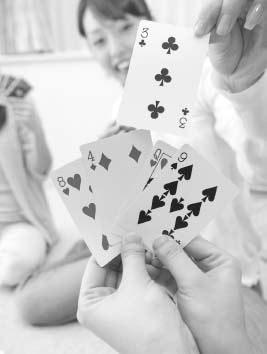NextPrevious

# How are probabilities of compound events determined?

Besides the probability of simple events, probabilities of compound events can also be computed. For example, if x and y represent two independent events, the probability that both x and y will occur is given by the product of their separate probabilities; and the probability that either of the two events will occur is given by the sum of their separate probabilities minus the probability that both will occur. For example, if the probability that a certain man will live to be 70 is 0.5, and the probability that his wife will live to be 70 is 0.6, the probability that they will both live to be 70 is 0.5 × 0.6 = 0.3; the probability that either the husband or wife will reach 70 is 0.5 + 0.6 - 0.3 = 0.8.The probability of drawing a particular suit from a pack of cards is 25 percent.

Close

This is a web preview of the "The Handy Math Answer Book" app. Many features only work on your mobile device. If you like what you see, we hope you will consider buying. Get the App